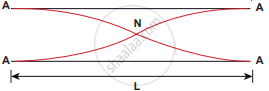Tamil Nadu Board of Secondary EducationHSC Science Class 11th

# Consider two organ pipes of the same length in which one organ pipe is closed and another organ pipe is open. If the fundamental frequency of closed pipe is 250 Hz. - Physics

Numerical

Consider two organ pipes of the same length in which one organ pipe is closed and another organ pipe is open. If the fundamental frequency of closed pipe is 250 Hz. Calculate the fundamental frequency of the open pipe.

#### SolutionClosed: L = lambda/4 => lambda = 4"L"Open: L = lambda/2 => lambda = 2"L"

Frequency of closed pipe (fc) = 250 Hz

Formula: "f"_0 = "V"/lambda

= "V"/(2"L")

"f"_0 = 2("V"/"4L")

= 2 "f"_"c"

= 2 × 250

Frequency of the open pipe = 500 Hz

Concept: Introduction to Waves
Is there an error in this question or solution?

Share